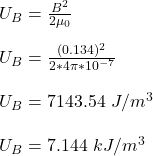## You would like to store 8.1 J of energy in the magnetic field of a solenoid. The solenoid has 620 circular turns of diameter 6.6 cm distribu

Question

You would like to store 8.1 J of energy in the magnetic field of a solenoid. The solenoid has 620 circular turns of diameter 6.6 cm distributed uniformly along its 33 cm length.
A. How much current is needed?
_____________ A
B. What is the magnitude of the magnetic field inside the solenoid?
________________T
C. What is the energy density (energy/volume) inside the solenoid?
________________ kJ/m^3

in progress 0
1 month 2021-08-13T20:55:27+00:00 1 Answers 0 views 0

(a) The current needed is 56.92 A

(b) The magnitude of the magnetic field inside the solenoid is 0.134 T

(c) The energy density inside the solenoid is 7.144 kJ/m³

Explanation:

Given;

energy stored in the magnetic field of solenoid, E = 8.1 J

number of turns of the solenoid, N = 620 turns

diameter of the solenoid, D = 6.6 cm = 0.066 m

radius of the solenoid, r = D/2 = 0.033 m

length of the solenoid, L = 33 cm = 0.33 m

Inductance of the solenoid is given as;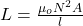where;

A is the area of the solenoid = πr² = π (0.033)² = 0.00342 m²

μ₀ is permeability of free space = 4π x 10⁻⁷ H/m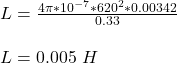(A). How much current needed

Energy stored in magnetic field of solenoid is given as;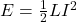Where;

I is the current in the solenoid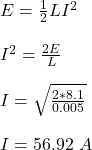(B) The magnitude of the magnetic field inside the solenoid

B = μ₀nI

where;

n is number of turns per unit length

B = μ₀(N/L)I

B = (4π x 10⁻⁷)(620/0.33)(56.92)

B = 0.134 T

(C) The energy density (energy/volume) inside the solenoid